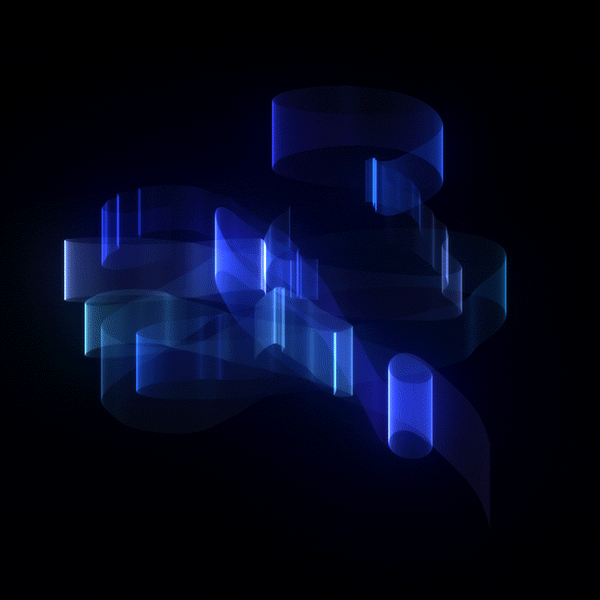E. Choroba github logo Apr 12・2 min readThis year's Google Code Jam Qualification round limit was 30 points. Solving just the two simpler tasks yielded enough to get you through.

I used Perl to solve the problems, as it's the language I can write the fastest.

You can find the problems in the Archive.

The first task sounded easy: the input is a sequence of numbers each containing the digit 4. For each number N, output two numbers A and B such that N = A + B and neither A nor B contains the digit 4.

I used the tr operator (similar to the `tr` utility you might know from *nix) to replace all 4's by 3's. That was my A. To get the B, I just subtracted A from the original N.

``````#! /usr/bin/perl
use warnings;
use strict;
use feature qw{ say };

chomp( my \$cases = <> );
for my \$case (1 .. \$cases) {
chomp( my \$n = <> );
(my \$i = \$n) =~ tr/4/3/;
my \$j = \$n - \$i;
say "Case #\$case: \$i \$j";
}
``````

This gave me the first 16 points. To get one more, it was needed to handle much larger numbers. It's possible by using the Math::BigInt core module, but I rather rushed to the next task.

``````use Math::BigInt;

...
my \$j = 'Math::BigInt'->new(\$n) - 'Math::BigInt'->new(\$i);
``````

You were given a path in a square where each step goes either to the south or to the east. You need to find a different path that starts and ends in the same places (which are the nortwest and southeast corners), but never reuses the given path.

The input path is encoded as a string like `SSEEESSE`. Surprisingly, the transliteration operator can again provide a short and straightforward solution. It corresponds to the path that's line symmetric with the input where the axis connects the start and end points.

``````#! /usr/bin/perl
use warnings;
use strict;
use feature qw{ say };

chomp( my \$cases = <> );
for my \$case (1 .. \$cases) {
<>;
chomp( my \$lydia_path = <> );
(my \$path = \$lydia_path) =~ tr/SE/ES/;
say "Case #\$case: \$path";
}
``````

This gave me 14 points, so my advancement was secured.

I also solved the third task, but the solution was ugly and didn't work for the larger inputs and extra points. It gave me 10 more points.

See you in the Round 1!

DISCUSS (1)dev.to now has dark mode.

Select night theme in the "misc" section of your settings ❤️I spent most of my time fighting compilation issues as they used super old TSC compiler (2.5) and it was not even configured properly. See you in round 1.

Classic DEV Post from Sep 7 '18

State of JavaScript 2018 Survey